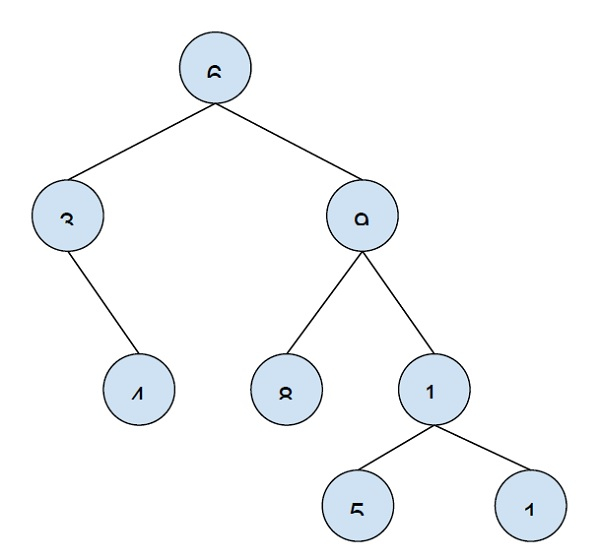# Print all nodes at distance k from a given node in C++

In this problem, we are given a binary tree, a target node and an integer K. We have to print all the nodes of the tree that are at a distance K from the target node.

Binary Tree is a special tree whose each node has at max two nodes (one/two/none).

Let’s take an example to understand the problemK = 2

Target node: 9

Output

5 1 3.

Explanation

The distance can be taken for node a higher, lower or at the same level. So, we will return nodes accordingly.

To solve this problem we have to understand what are the types of nodes that are K distance away from the target node.

From the above exam, we can see the k distant nodes can be in the subtree of the target node (5 and 1) or anywhere in the subtree of the ancestor of the target node(3).

Now, the method to find the solution of the first case is by traversing the subtree of the target node, and check if the distance of a node from the target is K. If yes print the node.

For the second case, we have to check the ancestor nodes and the subtree of these ancestors for the target and print all at distance K from it.

The below program will show the implementation of our solution −

## Example

Live Demo

#include <iostream>
using namespace std;
struct node {
int data;
struct node *left, *right;
};
void printSubtreeNodes(node *root, int k) {
if (root == NULL || k < 0) return;
if (k==0){
cout<<root->data<<"\t";
return;
}
printSubtreeNodes(root->left, k-1);
printSubtreeNodes(root->right, k-1);
}
int printKNodes(node* root, node* target , int k){
if (root == NULL) return -1;
if (root == target){
printSubtreeNodes(root, k);
return 0;
}
int dl = printKNodes(root->left, target, k);
if (dl != -1){
if (dl + 1 == k)
cout<<root->data<<"\t";
else
printSubtreeNodes(root->right, k-dl-2);
return 1 + dl;
}
int dr = printKNodes(root->right, target, k);
if (dr != -1){
if (dr + 1 == k)
cout << root->data << endl;
else
printSubtreeNodes(root->left, k-dr-2);
return 1 + dr;
}
return -1;
}
node *insertNode(int data){
node *temp = new node;
temp->data = data;
temp->left = temp->right = NULL;
return temp;
}
int main(){
node * root = insertNode(6);
root->left = insertNode(3);
root->right = insertNode(9);
root->left->right = insertNode(4);
root->right->left = insertNode(8);
root->right->right = insertNode(10);
root->right->right->left = insertNode(5);
root->right->right->right = insertNode(1);
node * target = root->right;
int K = 2;
cout<<"Nodes at distance "<<K<<" from the target node are :\n";
printKNodes(root, target, K);
return 0;
}

## Output

Nodes at distance 2 from the target n tode are −
5 1 3